Open in App
Not now

# Python OpenCV – Morphological Operations

• Last Updated : 03 Jan, 2023

Python OpenCV Morphological operations are one of the Image processing techniques that processes image based on shape. This processing strategy is usually performed on binary images.

Morphological operations based on OpenCV are as follows:

• Erosion
• Dilation
• Opening
• Closing
• Top hat
• Black hat

For all the above techniques the two important requirements are the binary image and a kernel structuring element that is used to slide across the image.

## Images used for demonstration: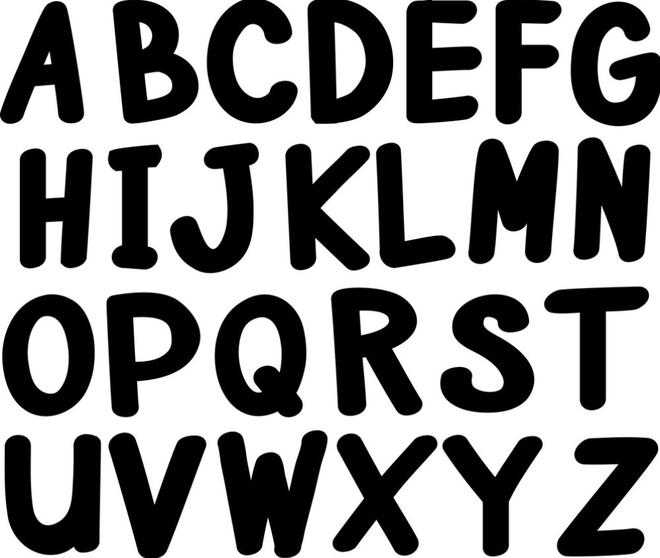Images used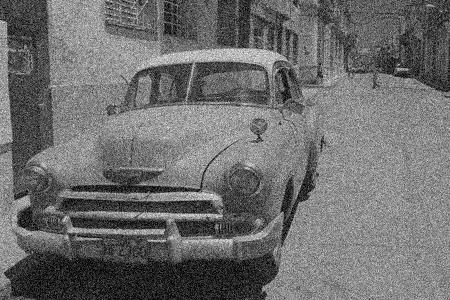Images used

## Erosion

Erosion primarily involves eroding the outer surface (the foreground) of the image. As binary images only contain two pixels 0 and 255, it primarily involves eroding the foreground of the image and it is suggested to have the foreground as white. The thickness of erosion depends on the size and shape of the defined kernel. We can make use of NumPy’s ones() function to define a kernel. There are a lot of other functions like NumPy zeros, customized kernels, and others that can be used to define kernels based on the problem in hand.

Code:

• Import the necessary packages as shown
• Binarize the image.
• As it is advised to keep the foreground in white, we are performing OpenCV’s invert operation on the binarized image to make the foreground as white.
• We are defining a 5×5 kernel filled with ones
• Then we can make use of Opencv erode() function to erode the boundaries of the image.

## Python3

 `# import the necessary packages ` `import` `cv2 ` `import` `numpy as np ` `import` `matplotlib.pyplot as plt ` ` `  `# read the image ` `img ``=` `cv2.imread(r``"Downloads\test (2).png"``, ``0``) ` ` `  `# binarize the image ` `binr ``=` `cv2.threshold(img, ``0``, ``255``, cv2.THRESH_BINARY``+``cv2.THRESH_OTSU)[``1``] ` ` `  `# define the kernel ` `kernel ``=` `np.ones((``5``, ``5``), np.uint8) ` ` `  `# invert the image ` `invert ``=` `cv2.bitwise_not(binr) ` ` `  `# erode the image ` `erosion ``=` `cv2.erode(invert, kernel, ` `                    ``iterations``=``1``) ` ` `  `# print the output ` `plt.imshow(erosion, cmap``=``'gray'``) `

Output:

The output should be a thinner image than the original one.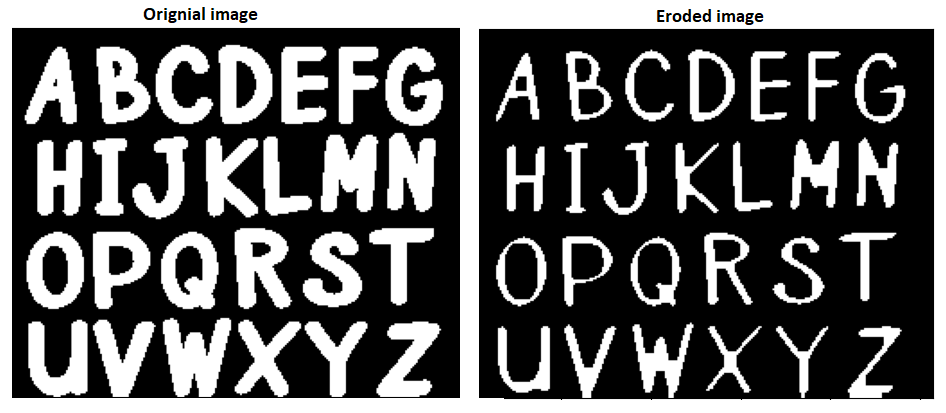Erosion

## Dilation

Dilation involves dilating the outer surface (the foreground) of the image. As binary images only contain two pixels 0 and 255, it primarily involves expanding the foreground of the image and it is suggested to have the foreground as white. The thickness of erosion depends on the size and shape of the defined kernel. We can make use of NumPy’s ones() function to define a kernel. There are a lot of other functions like NumPy zeros, customized kernels, and others that can be used to define kernels based on the problem at hand. It is exactly opposite to the erosion operation

Code:

• Import the necessary packages as shown
• Binarize the image.
• As it is advised to keep the foreground in white, we are performing OpenCV’s invert operation on the binarized image to make the foreground white.
• We are defining a 3×3 kernel filled with ones
• Then we can make use of the Opencv dilate() function to dilate the boundaries of the image.

## Python3

 `import` `cv2 ` ` `  `# read the image ` `img ``=` `cv2.imread(r``"path to image"``, ``0``) ` ` `  `# binarize the image ` `binr ``=` `cv2.threshold(img, ``0``, ``255``, cv.THRESH_BINARY``+``cv.THRESH_OTSU)[``1``] ` ` `  `# define the kernel ` `kernel ``=` `np.ones((``3``, ``3``), np.uint8) ` ` `  `# invert the image ` `invert ``=` `cv2.bitwise_not(binr) ` ` `  `# dilate the image ` `dilation ``=` `cv2.dilate(invert, kernel, iterations``=``1``) ` ` `  `# print the output ` `plt.imshow(dilation, cmap``=``'gray'``) `

Output:

The output should be a thicker image than the original one.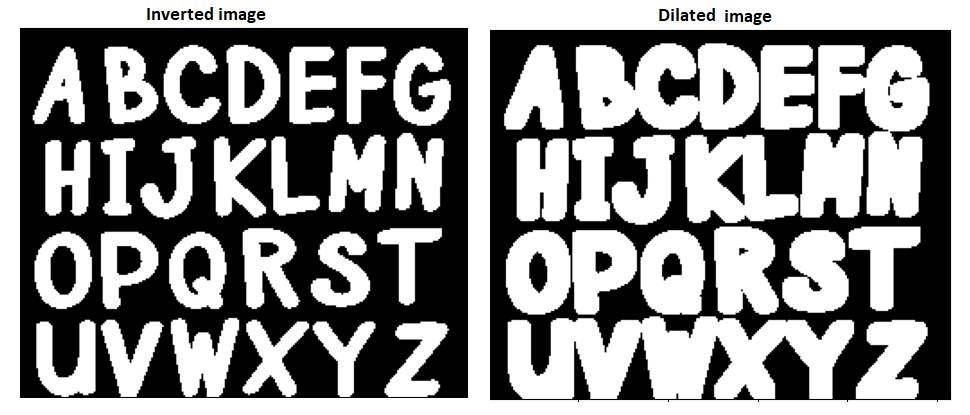Dilated image

## Opening

Opening involves erosion followed by dilation in the outer surface (the foreground) of the image. All the above-said constraints for erosion and dilation applies here. It is a blend of the two prime methods. It is generally used to remove the noise in the image.

Code:

• Import the necessary packages as shown
• Binarize the image.
• We are defining a 3×3 kernel filled with ones
• Then we can make use of the Opencv cv.morphologyEx() function to perform an Opening operation on the image.

## Python3

 `# import the necessary packages ` `import` `cv2 ` ` `  `# read the image ` `img ``=` `cv2.imread(r``"\noise.png"``, ``0``) ` ` `  `# binarize the image ` `binr ``=` `cv2.threshold(img, ``0``, ``255``, ` `                     ``cv2.THRESH_BINARY``+``cv2.THRESH_OTSU)[``1``] ` ` `  `# define the kernel ` `kernel ``=` `np.ones((``3``, ``3``), np.uint8) ` ` `  `# opening the image ` `opening ``=` `cv2.morphologyEx(binr, cv2.MORPH_OPEN, ` `                           ``kernel, iterations``=``1``) ` `# print the output ` `plt.imshow(opening, cmap``=``'gray'``) `

Output: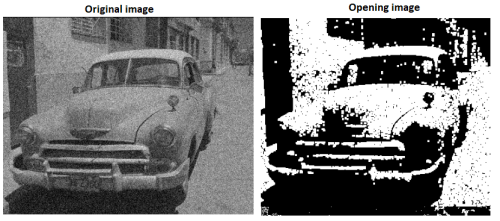Opening Image

## Closing

Closing involves dilation followed by erosion in the outer surface (the foreground) of the image. All the above-said constraints for erosion and dilation applies here. It is a blend of the two prime methods. It is generally used to remove the noise in the image.

Code:

• Import the necessary packages as shown
• Binarize the image.
• We are defining a 3×3 kernel filled with ones
• Then we can make use of the Opencv cv.morphologyEx() function to perform a Closing operation on the image.

## Python3

 `# import the necessary packages ` `import` `cv2 ` ` `  `# read the image ` `img ``=` `cv2.imread(r``"\Images\noise.png"``, ``0``) ` ` `  `# binarize the image ` `binr ``=` `cv2.threshold(img, ``0``, ``255``, cv2.THRESH_BINARY``+``cv2.THRESH_OTSU)[``1``] ` ` `  `# define the kernel ` `kernel ``=` `np.ones((``3``, ``3``), np.uint8) ` ` `  `# opening the image ` `closing ``=` `cv2.morphologyEx(binr, cv2.MORPH_CLOSE, kernel, iterations``=``1``) ` ` `  `# print the output ` `plt.imshow(closing, cmap``=``'gray'``) `

Output: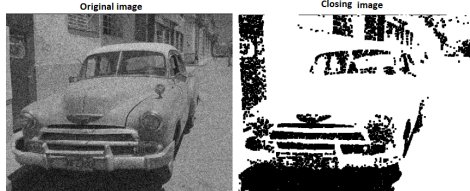Closing Image

Morphological gradient is slightly different than the other operations, because, the morphological gradient first applies erosion and dilation individually on the image and then computes the difference between the eroded and dilated image. The output will be an outline of the given image.

Code:

• Import the necessary packages as shown
• Binarize the image.
• As it is advised to keep the foreground in white, we are performing OpenCV’s invert operation on the binarized image to make the foreground as white.
• We are defining a 3×3 kernel filled with ones
• Then we can make use of the Opencv cv.morphologyEx() function to perform a Morphological gradient on the image.

## Python3

 `# import the necessary packages ` `import` `cv2 ` ` `  `# read the image ` `img ``=` `cv2.imread(r``"path to your image"``, ``0``) ` ` `  `# binarize the image ` `binr ``=` `cv2.threshold(img, ``0``, ``255``, cv2.THRESH_BINARY``+``cv2.THRESH_OTSU)[``1``] ` ` `  `# define the kernel ` `kernel ``=` `np.ones((``3``, ``3``), np.uint8) ` ` `  `# invert the image ` `invert ``=` `cv2.bitwise_not(binr) ` ` `  `# use morph gradient ` `morph_gradient ``=` `cv2.morphologyEx(invert, ` `                                  ``cv2.MORPH_GRADIENT,  ` `                                  ``kernel) ` ` `  `# print the output ` `plt.imshow(morph_gradient, cmap``=``'gray'``) `

Output: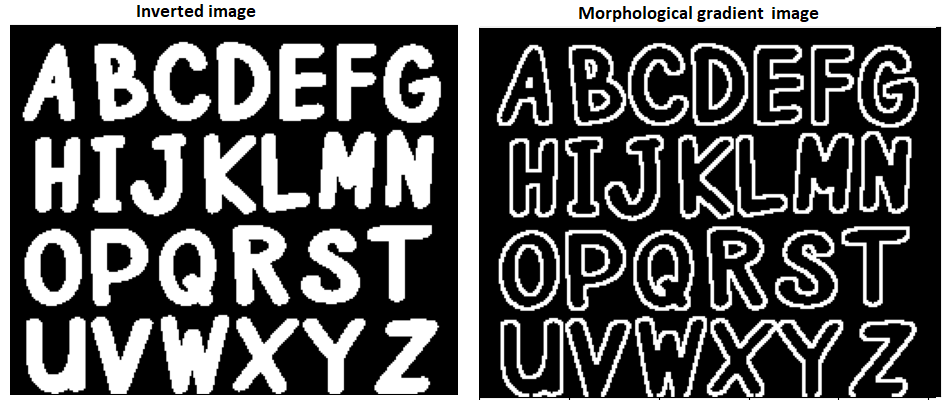## Top Hat

Top Hat is yet another morphological operation where Opening is performed on the binary image and the output of this operation is a difference between the input image and the opened image.

Code:

• Import the necessary packages as shown.
• Binarize the image.
• We are defining a 13×13 kernel filled with ones.
• Then we can make use of the Opencv cv.morphologyEx() function to perform a Top Hat operation on the image.

## Python3

 `# import the necessary packages ` `import` `cv2 ` ` `  `# read the image ` `img ``=` `cv2.imread(``"your image path"``, ``0``) ` ` `  `# binarize the image ` `binr ``=` `cv2.threshold(img, ``0``, ``255``, cv2.THRESH_BINARY``+``cv2.THRESH_OTSU)[``1``] ` ` `  `# define the kernel ` `kernel ``=` `np.ones((``13``, ``13``), np.uint8) ` ` `  `# use morph gradient ` `morph_gradient ``=` `cv2.morphologyEx(binr, ` `                                  ``cv2.MORPH_TOPHAT, ` `                                  ``kernel) ` `# print the output ` `plt.imshow(morph_gradient, cmap``=``'gray'``) `

Output: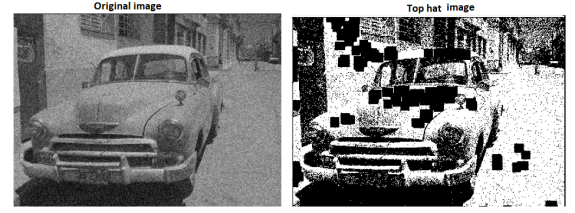Top hat Image

## Black Hat

The black-hat operation is used to do the opposite, enhancing dark objects of interest on a bright background. The output of this operation is the difference between the closing of the input image and the input image.

Code:

• Import the necessary packages as shown.
• Binarize the image.
• As it is advised to keep the foreground white, we are performing OpenCV’s invert operation on the binarized image to make the foreground as white.
• We are defining a 5×5 kernel filled with ones.
• Then we can use the Opencv cv.morphologyEx() function to perform a Top Hat operation on the image.

## Python3

 `# import the necessary packages ` `import` `cv2 ` ` `  `# read the image ` `img ``=` `cv2.imread(``"your image path"``, ``0``) ` ` `  `# binarize the image ` `binr ``=` `cv2.threshold(img, ``0``, ``255``, cv2.THRESH_BINARY``+``cv2.THRESH_OTSU)[``1``] ` ` `  `# define the kernel ` `kernel ``=` `np.ones((``5``, ``5``), np.uint8) ` ` `  `# invert the image ` `invert ``=` `cv2.bitwise_not(binr) ` ` `  `# use morph gradient ` `morph_gradient ``=` `cv2.morphologyEx(invert, ` `                                  ``cv2.MORPH_BLACKHAT, ` `                                  ``kernel) ` `# print the output ` `plt.imshow(morph_gradient, cmap``=``'gray'``) `

Output: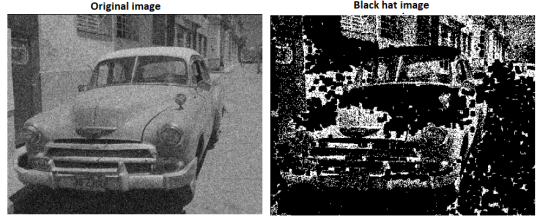Black Hat image

My Personal Notes arrow_drop_up
Related Articles